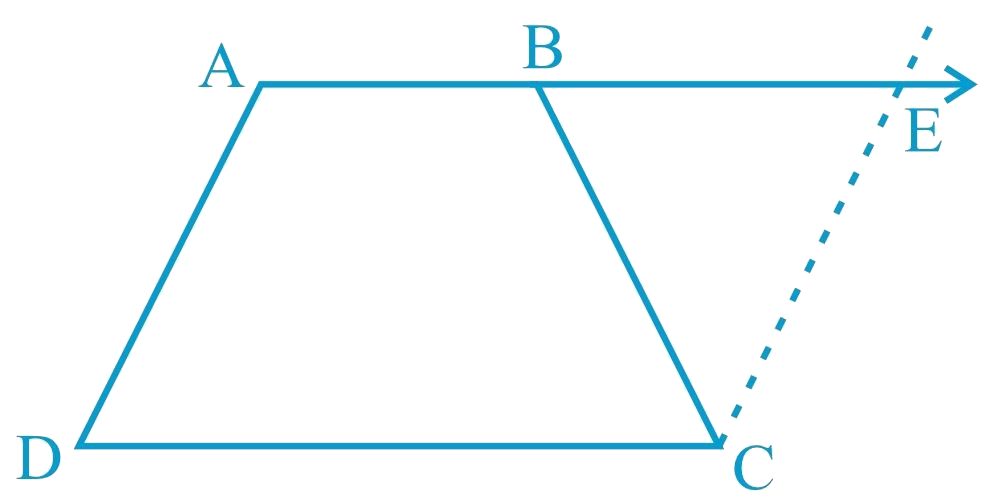In the verge of coronavirus pandemic, we are providing FREE access to our entire Online Curriculum to ensure Learning Doesn't STOP!

# Ex.8.1 Q12 Quadrilaterals Solution - NCERT Maths Class 9

Go back to  'Ex.8.1'

## Question

$$ABCD$$ is a trapezium in which $$AB\; ||\; CD$$ and $$AD = BC$$ (see the given figure). Show that(i) \begin{align} &{ \angle {A}=\angle {B}} \end{align}

(ii) \begin{align} & { \angle {C}=\angle {D}} \end{align}

(iii) \begin{align}&{ \triangle {ABC} \cong \triangle {BAD}} \end{align}

(iv) diagonal $$AC =$$ diagonal $$BD$$

[Hint: Extend $$AB$$ and draw a line through $$C$$ parallel to $$DA$$ intersecting $$AB$$ produced at $$E$$.]

Video Solution
Ex 8.1 | Question 12

## Text Solution

What is known?

$$ABCD$$ is a trapezium in which $$AB || CD$$ and $$AD = BC.$$

What is unknown?

How we can show that

(i) $$∠A = ∠B$$

(ii) $$∠C = ∠D$$

(iii) $$∆ABC ≅ ∆BAD$$

(iv) diagonal $$AC =$$ diagonal $$BD$$

Reasoning:

Consider parallel lines $$AD$$ and $$CE, AE$$ is the transversal line for them then sum of co-interior angles will be $$180$$ degree also angles $$CBE$$ and $$CBA$$ are linear pairs gives sum $$180$$, angles $$CEB$$ equals to $$CBE$$ because opposite to equal sides in triangle $$BCE$$. Using these observations angle A is equal to angle B. Then AB is parallel to CD will help to show angle C is equal to angle D. Also, by using suitable congruence criterion we can show triangles congruent then we can say corresponding parts of congruent triangles will be equal.

Steps:

Let us extend $$AB$$. Then, draw a line through $$C$$, which is parallel to $$AD$$, intersecting $$AB$$ at point $$E$$. It is clear that $$AECD$$ is a parallelogram.

(i) $$AD = CE$$ (Opposite sides of parallelogram $$AECD$$)

However, $${AD}={BC}(\text { Given })$$

Therefore, $${BC}={CE}$$

\begin{align}&\angle {{CEB}} = \angle {{CBE}}\\&\left( \begin{array}{l}{\text{Angle opposite to equal }}\\{\text{sides are also equal}}\end{array} \right)\end{align}

Consider parallel lines  and .  is the transversal line for them.

\begin{align}&\angle {{A}} + \angle {{CEB}} = {180^\circ }\\&\left( \begin{array}{l}{\text{Angles on the same }}\\{\text{side of transversal}}\end{array} \right)\end{align}

\begin{align}&\angle {{A}} + \angle {{CBE}} = {180^\circ }\\&\left( \begin{array}{l}{\text{Using the relation }}\\\angle {{CEB}} = \angle {{CBE}}\end{array} \right) \ldots (1)\end{align}

However, $$\angle B$$ + $$\angle CBE$$ = (Linear pair angles) ... (2)

From Equations (1) and (2), we obtain

$$\angle A$$ = $$\angle B$$

(ii)

\begin{align}&\angle A + \angle D = {180^\circ }\\&\left( \begin{array}{l}{\text{Angles on the same side}}\\{\text{ of the transversal}}\end{array} \right)\end{align}

Also,
\begin{align}&\angle C + \angle B = {180^\circ }\\&\left( \begin{array}{l}{\text{Angles on the same side }}\\{\text{of the transversal}}\end{array}\right)\end{align}

$\therefore \angle A + \angle D = \angle C + \angle B$

However,
\begin{align}&\angle A = \angle B\\&\left[ \begin{array}{l}{\text{Using the result }}\\{\text{obtained in (i) }}\end{array} \right]\\&\therefore \,\,\,\angle C = \angle D\end{align}

(iii) In \begin{align}\triangle {ABC} \text { and } \triangle {BAD}\end{align}

\begin{align}{{AB}} &= {{BA \text{(Common side) }}}\\{{BC}}& = AD\text {(Given) }\\\angle {{B}} &= \angle A {\text{(Proved before) }}\\\therefore \Delta {{ABC}} &\cong \Delta {{BAD }}\\&\left( {{\text{SAS congruence rule}}} \right)\end{align}

\begin{align} \Delta {ABC}& \cong \Delta {BAD} \\ \;\;\;\;\therefore {AC}&= {{BD}({By} \;{CPCT})}\end{align}